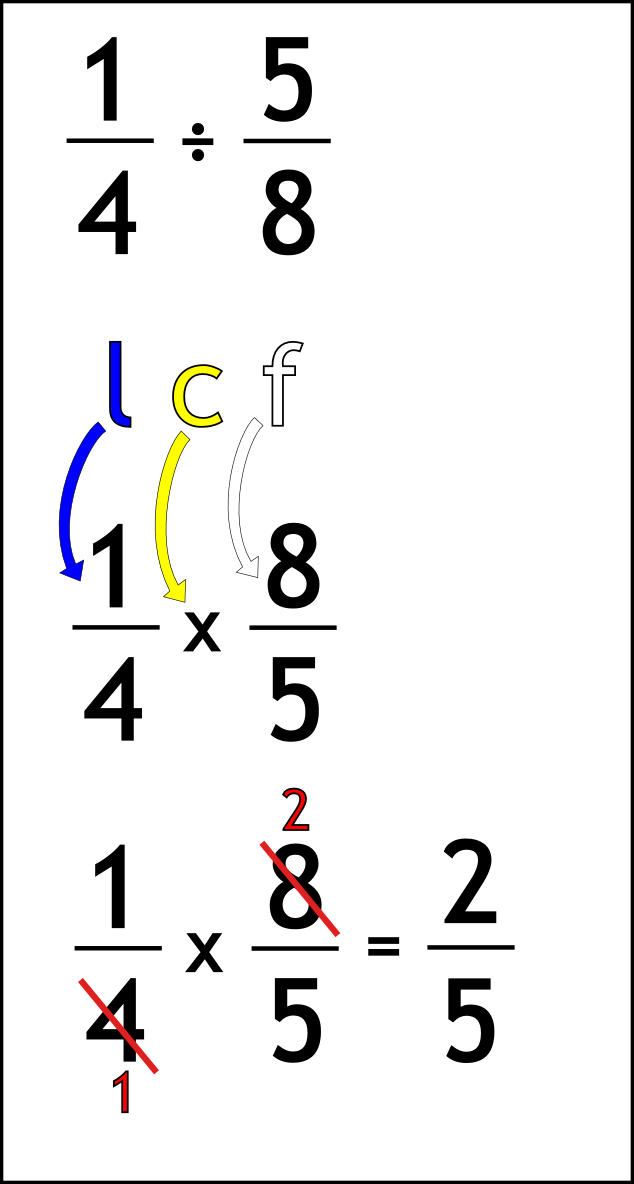The Singing Hedgehog Guide to:
Dividing Fractions 1

We call these questions

FLIPPIN' DIVISION!

The magic phrase to remember is:

LEAVE IT, CHANGE IT, FLIP IT!

Here is a question:Write out the question

Do the magic phrase:
leave the first fraction
change the sign to times

flip the second fraction

Now we just have a times question to do
Find common factors (numbers to divide by)

1 and 5 can't simplify further so we leave them

4 and 8 are both divisible by 2 to leave 1 and 2

Multiply across

next page

fractions index